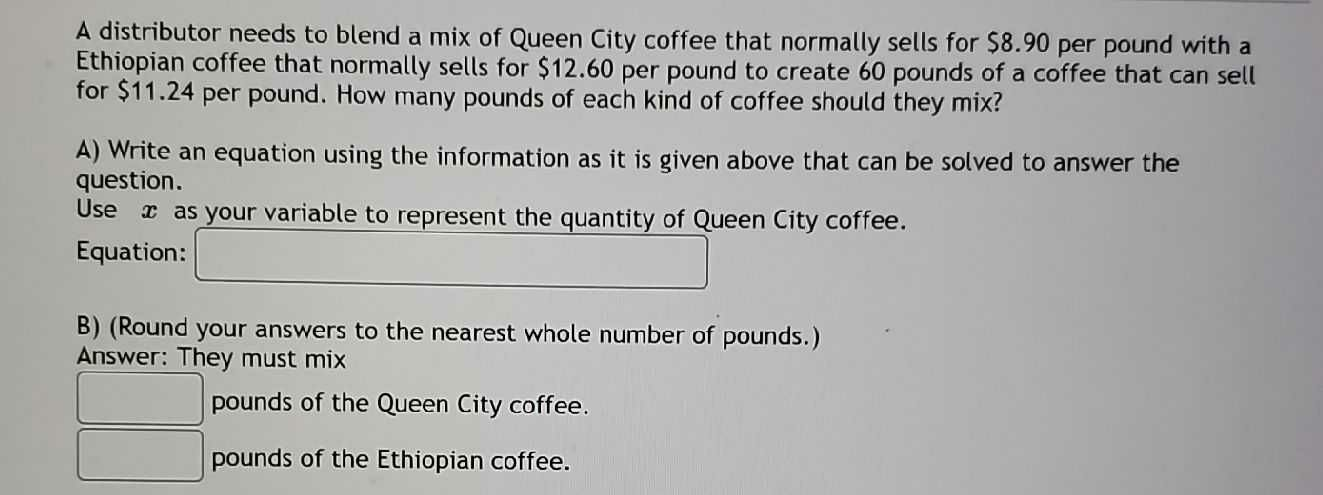### ¿Todavía tienes preguntas de matemáticas?

Pregunte a nuestros tutores expertos
Algebra
PreguntaA distributor needs to blend a mix of Queen City coffee that normally sells for $$\ 8.90$$ per pound with a Ethiopian coffee that normally sells for $$\ 12.60$$ per pound to create $$60$$ pounds of a coffee that can sell for $$\ 11.24$$ per pound. How many pounds of each kind of coffee should they mix?

A) Write an equation using the information as it is given above that can be solved to answer the question. Use $$x$$ as your variable to represent the quantity of Queen City coffee. Equation:_______

_______pounds of the Queen City coffee.

________pounds of the Ethiopian coffee.